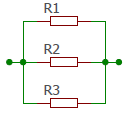# Resistors in parallel

Calculator and formulas for calculating resistors in parallel

## Calculate resistance parallel connection

When resistors are connected in parallel, the current is distributed over the individual resistors.## Calculate total resistance

Exponents are not allowed. Enter all values in a suitable, equal unit of measurement. If you enter all values, e.g. in kOhm, the result is also displayed in kOhm.

For the calculation, enter the values of the individual resistors separated by a semicolon.
Example: 33; 12,1; 22

Resistors in parallel calculator

 Enter the resistances separated by semicolonst Decimal places 0 1 2 3 4 6 8 10 Result Total resistance:

## Formulas for resistors in parallel

In order to calculate the total resistance of several resistors in parallel, their conductance values are added. The conductance is the reciprocal of the resistance. The formula for three resistors connected in parallel is:

$$\displaystyle \frac{1}{R_{ges}}=\frac{1}{R_1}+\frac{1}{R_2}+\frac{1}{R_3}$$

If the total resistance of two parallel resistors is to be calculated, the following formula can be used.

$$\displaystyle R_{ges}=\frac{R_1· R_2}{R_1 + R_2}$$